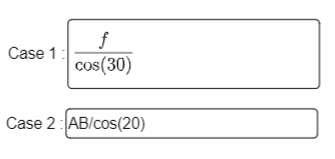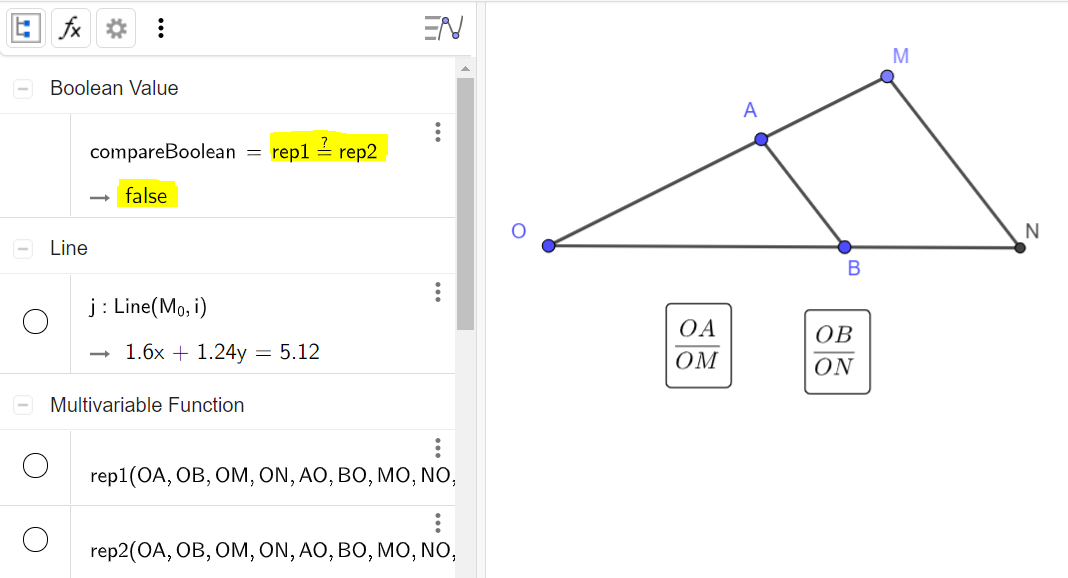# Algebra - New version ?

frpiou shared this problem 2 years ago
Not a Problem

Hi !

Before (a several month ago) i did'nt have a problem with this but now AB isn't interpret by GeoGebra. Is it a mystake ? What is the point ?Cumbersome, but maybe we can make it.

Rename the vertices of the segment as e.g. P1 and P2, but show their labels as A and B.

Rename the segment as g, but display its label as f.

Create a 2 variables function for the input box, like e.g.

rep2_{1}(AB, f) = f cos(30°) + AB

Then enter SetValue(rep2_{1},?) to set it as undefined, but still be a function in 2 variables.

Then try with numerical testing to see if the student entry was correct.

Can this work?

See attached file, and try to enter in the 2nd input box terms containing AB or f.1

In an InputBox, when the name of a variable have more than one letter, GeoGebra doesn't interpret correctly this but before it was not a problem. It was even possible to directly write "Distance(A,B)".1

Yes, we've "locked down" Input Boxes a bit recently1

But all my work is broken now... How can i do ?1

Sorry, I don't have a good suggestion for you. It's possible to use an older version like this: https://wiki.geogebra.org/e...1

Unfortunately not, my work is used only online.But my question is, why not keeping this functionality ? Is it definitely ?1

as poor workaround you can rename AB to D_AB or A_B and type it in inputbox

or to use a text for input and a script with parsetonumber()1

Thank you but my aim is to let students write the distance between two points in a usual mathematical notation.1

You can use it with a script:

case2 InputBox(a)

a="" -Text

Execute[{"rep2="a }] -set in AB or in Segment f https://www.geogebra.org/ma...1

You can use it with a script1

Thank you Roman Chijner but it is text and i want number to have a symbolic interpretation like f/cos(30) in the example below.1

Thank you Roman Chijner but it is text and i want number to have a symbolic interpretation like f/cos(30) in the example below.1

Cumbersome, but maybe we can make it.

Rename the vertices of the segment as e.g. P1 and P2, but show their labels as A and B.

Rename the segment as g, but display its label as f.

Create a 2 variables function for the input box, like e.g.

rep2_{1}(AB, f) = f cos(30°) + AB

Then enter SetValue(rep2_{1},?) to set it as undefined, but still be a function in 2 variables.

Then try with numerical testing to see if the student entry was correct.

Can this work?

See attached file, and try to enter in the 2nd input box terms containing AB or f.1

rep2_{1} seems to work fine except it shows red dot border when editing1

@Simone Riva

It is excellent ! Thank you for this solution ! https://www.geogebra.org/cl...

There is another problem : with this solution i can't compare rep1 and rep2 like i usually do :1

@mathmagic Subscripts are usually well recognized if enclosed in braces. Now ggb shows a dotted input box when there is an invalid input, so for example if I enter an x for a function that is defined depending on other variables than x.

But I usually avoid subscripts like the plague...1

You can compare the texts of the two input boxes, and set the Boolean “correct” when the first is OA/OM or OB/ON && the second text is OA/OM or OB/ON && the 2nd text is not equal to the first one.

Not very robust, but it should work. Use command Text to get the text of the content of each input box.

Or you might use numerical testing: the two functions must yield the same results when evaluated at a point. Choosing the coordinates of this point wisely.

And I’m not sure that using just one test point is enough in this case, but it’s 00:20 and my two neurons are disconnecting from each other 😂1

Thanks you so much !

I solved the last problem with ParseToNumber() function.

Here is the complete solution :

First i define my Input boxes with symbolic option for boths :

```rep1(OA,OB,OM,ON,AO,BO,MO,NO,AM,MA,BN,NB,AB,BA,MN,NM)=?
rep2(OA,OB,OM,ON,AO,BO,MO,NO,AM,MA,BN,NB,AB,BA,MN,NM)=?
InputBox1=InputBox(k_1)
InputBox1=InputBox(k_2)
```

Then i put the responses in text (your suggestion)

```text1=Text(rep1)
text2=Text(rep2)```

I define every distances (with your suggestion)

```OA=Distance(O_0,A_0)
OB=Distance(O_0,B_0)
ON=Distance(O_0,N_0)
OM=Distance(O_0,M_0)
AB=Distance(A_0,B_0)
MN=Distance(M_0,N_0)
etc.```
I create two number to evaluate the responses :
```k_1
k_2```

And a boolean to test the equality

`compare=k_1==k_2`

I create a button with this code

```ParseToNumber(k_1,text1)
ParseToNumber(k_2,text2)```
The only problem is that the two number k_1 and k_2 are with 5 digits.

It could make wrong answers sometimes.